# Mathematics software reviews

Showing 101 to 114 from 114 matches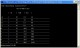Horny - Das Programm zum Horner Schema 1.2 Mathematics FreewareMath-Flight 2 2.3 Mathematics Fly High while having fun learning basic mathematics with Math Flight! Shareware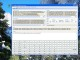Complex Calculator Precision 36 1.0.1.1 Mathematics Complex number calculator for scientists, engineers, teachers, and students. Shareware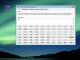Compact Scientific Calculator 45 1.0.1.1 Mathematics Scientific calculator for scientists, engineers, teachers, and students. Shareware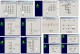Divide Whole Numbers 3.3 Mathematics Whole number and fraction computation. Number by number problem exercises. DemoInternetMath 7 Mathematics Uses the Internet to teach how to solve math word problems grades 1-8 SharewareAdd & Subtract Whole Numbers 3.2 Mathematics Whole number and fraction computation. Number by number problem exercises. DemoAbilities Builder Math Facts 6.5 Mathematics Whole number and fraction computation. Number by number problem exercises. DemoMuptiply Whole Numbers 6.7 Mathematics Whole number and fraction computation. Number by number problem exercises. Demo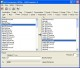UnitConvertor-A 2.4.16 Mathematics Professional and comprehensive unit-converter for Windows Shareware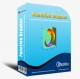Function Grapher 3.9.2 Mathematics Graph maker to create 2d, 2.5d, 3D and 4d function graphs and animations. Demo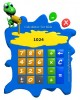Calculator for Kids 1.0 Mathematics Simple calculator for kids including basic math operations: addition, subtractio Freeware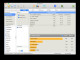AcaStat Mac 10.5.2 Mathematics Inexpensive and easy-to-use statistical tool. Mac and Windows. DemoCurveFitter 4.5.22 Mathematics CurveFitter performs regression analysis to estimate values of parameters. Shareware